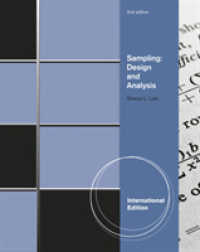### Sampling : Design and Analysis, International Edition -- Paperback （Internatio）

• ただいまウェブストアではご注文を受け付けておりません。 ⇒古書を探す
• 製本 Paperback:紙装版/ペーパーバック版
• 商品コード 9780495110842

### Full Description

Sharon L. Lohr's SAMPLINGprovides a modern introduction to the field of survey sampling intended for a wide audience of statistics students. Practical and authoritative, the book is listed as a standard reference for training on real-world survey problems by a number of prominent surveying organizations. Lohr concentrates on the statistical aspects of taking and analyzing a sample, incorporating a multitude of applications from a variety of disciplines. The text gives guidance on how to tell when a sample is valid or not, and how to design and analyze many different forms of sample surveys. Recent research on theoretical and applied aspects of sampling is included, as well as optional technology instructions for using statistical software with survey data.

### Contents

1. INTRODUCTION.A Sample Controversy. Requirements of a Good Sample. Selection Bias. Measurement Error. Questionnaire Design. Sampling and Nonsampling Errors. Exercises. 2. SIMPLE PROBABILITY SAMPLES. Types of Probability Samples. Framework for Probability Sampling. Simple Random Sampling. Sampling Weights. Confidence Intervals. Sample Size Estimation. Systematic Sampling. Randomization Theory Results for Simple Random Sampling. A Prediction Approach for Simple Random Sampling. When Should a Simple Random Sample Be Used? Chapter Summary. Exercises.3. STRATIFIED SAMPLING. What Is Stratified Sampling? Theory of Stratified Sampling. Sampling Weights in Stratified Random Sampling. Allocating Observations to Strata. Defining Strata. Model-Based Inference for Stratified Sampling. Quota Sampling.Chapter Summary. Exercises.4. RATIO AND REGRESSION ESTIMATION.Ratio Estimation in a Simple Random Sample. Estimation in Domains.Regression Estimation in Simple Random Sampling. Poststratification.Ratio Estimation with Stratified Samples. Model-Based Theory for Ratio and Regression Estimation. Chapter Summary. Exercises.5. CLUSTER SAMPLING WITH EQUAL PROBABILITIES. Notation for Cluster Sampling. One-Stage Cluster Sampling. Two-Stage Cluster Sampling. Designing a Cluster Sample. Systematic Sampling. Model-Based Inference in Cluster Sampling. Chapter Summary. Exercises. 6. SAMPLING WITH UNEQUAL PROBABILITIES. Sampling One Primary Sampling Unit. One-Stage Sampling with Replacement.Two-Stage Sampling with Replacement. Unequal Probability Sampling Without Replacement. Examples of Unequal Probability Samples. Randomization Theory Results and Proofs. Models and Unequal Probability Sampling. Chapter Summary.Exercises. 7. COMPLEX SURVEYS. Assembling Design Components. Sampling Weights. Estimating a Distribution Function. Plotting Data from a Complex Survey. Univariate Plots. Design Effects. The National Crime Victimization Survey. Sampling and Experiment Design.Chapter Summary. Exercises. 8. NONRESPONSE. Effects of Ignoring Nonresponse. Designing Surveys to Reduce Nonsampling Errors. Callbacks and Two-Phase Sampling. Mechanisms for Nonresponse. Weighting Methods for Nonresponse. Imputation. Parametric Models for Nonresponse. What Is an Acceptable Response Rate? Chapter Summary. Exercises. Linearization (Taylor Series) Methods. Random Group Methods. Resampling and Replication Methods. Generalized Variance Functions. Confidence Intervals.Chapter Summary. Exercises. Chi-Square Tests with Multinomial Sampling. Effects of Survey Design on Chi-Square Tests. Corrections to x2 Tests. Loglinear Models. Chapter Summary. Exercises. 11. REGRESSION WITH COMPLEX SURVEY DATA. Model-Based Regression in Simple Random Samples. Regression in Complex Surveys. Should Weights Be Used in Regression? Mixed Models for Cluster Samples. Logistic Regression. Generalized Regression Estimation for Population Totals. Chapter Summary. Exercises.12. TWO-PHASE SAMPLING. Theory for Two-Phase Sampling. Two-Phase Sampling with Stratification. Two-Phase Sampling with Ratio Estimation. Subsampling Nonrespondents. Designing a Two-Phase Sample. Chapter Summary. Exercises. 13. ESTIMATING POPULATION SIZE. Capture-Recapture Estimates. Contingency Tables for Capture-Recapture Experiments. Assessing Undercoverage. Chapter Summary. Exercises.14. RARE POPULATIONS AND SMALL AREA ESTIMATIONS.Sampling for Rare Events. Small Area Estimation. Chapter Summary. Exercises. 15. SURVEY QUALITY. Nonresponse Error. Measurement Error. Sensitive Questions. Processing Error. Sampling Error. Interaction of Error Sources. The Future of Sampling. Chapter Summary. Exercises. Probability. Random Variables and Expected Value. Conditional Probability. Conditional Expectation. REFERENCES.